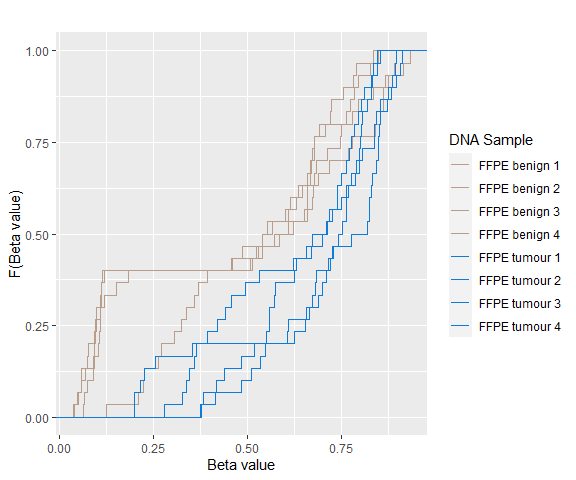# Introduction

The methylation state of a cytosine-guanine dinucleotide (CpG) site is hypermethylated if both the alleles are methylated, hypomethylated if neither of the alleles are methylated and hemimethylated otherwise. A differentially methylated CpG (DMC) site has different methylation states between different samples. Identifying the DMCs between benign and malignant tissue samples can help understand disease and its treatment. The methylation values are known as beta values and can be modelled using beta distributions. The beta values are constrained between 0 and 1 and a beta value close to 0 suggests hypomethylation whereas a value close to 1 suggests hypermethylation. Due to a lack of suitable methods for the beta values in their innate form, beta values are usually transformed to M-values, which can be modelled using a Gaussian distribution. The DMCs are identified using M-values or beta values via multiple t-tests but this can be computationally expensive. Also, arbitrary thresholds are selected to identify the methylation states and used to identify the methylation state of a CpG site.

The package betaclust contains a family of novel beta mixture models (BMMs) which use a model-based clustering approach to cluster the CpG sites in their innate beta form to (i) objectively identify methylation state thresholds and (ii) identify the DMCs between different samples. The family of BMMs employs different parameter constraints applicable to different study settings. The EM algorithm is used for parameter estimation, with a novel approximation during the M-step providing tractability and ensuring computational feasibility.

This document gives a quick tour of the functionalities in betaclust. See help(package="betaclust") for further details and references provided by citation("betaclust").

# Walk through

## Prerequisites

Before starting the betaclust walk through, the user should have a working R software environment installed on their machine. The betaclust package has the following dependencies which, if not already installed on the machine will automatically be installed along with the package: foreach, doParallel, stats, utils, ggplot2, plotly, scales, devtools.

Assuming that the user has the betaclust package installed, the user first needs to load the package:

library(betaclust)

The betaclust package provides a preprocessed methylation dataframe which contains beta values of DNA samples collected from 4 patients suffering from high-grade prostate cancer. The samples are collected from benign and tumor prostate tissues. The methylation profiling of these samples is done using the Infinium MethylationEPIC Beadchip technology. The dataset comprises R = 2 DNA samples collected from each of N = 4 patients and each sample contains beta values at C = 694,820 CpG sites. The data were collected for a study on prostate cancer methylomics (Silva et al. 2020).

The methylation array data was quality controlled and preprocessed using the RnBeads package (Mueller et al. 2019). The data were then normalized and probes located outside of CpG sites and on the sex chromosome were filtered out. The CpG sites with missing values were removed from the resulting dataset. A subset of the complete PCa dataset (Majumdar et al. 2022) with C = 5,067 CpG sites is available in the package for testing purposes. The user can load the data available in the package and look at the first 6 rows present in the dataframe as follows:

data(pca.methylation.data)
#>          IlmnID FFPE_benign_1 FFPE_benign_2 FFPE_benign_3 FFPE_benign_4
#> 2    cg26928153    0.89455232    0.91409788    0.84163758    0.93078887
#> 141  cg04531633    0.03208166    0.08281574    0.05740011    0.02988679
#> 695  cg13924635    0.92546986    0.89308795    0.94957052    0.97076451
#> 878  cg16527939    0.38656364    0.37669476    0.45448635    0.36087991
#> 885  cg26831087    0.64713166    0.62123119    0.80137693    0.64403769
#> 1026 cg22389936    0.91281793    0.94080964    0.91642979    0.93504925
#>      FFPE_tumour_1 FFPE_tumour_2 FFPE_tumour_3 FFPE_tumour_4
#> 2       0.87535211    0.95826738    0.91968680    0.93482428
#> 141     0.09634015    0.07898189    0.06564365    0.04237288
#> 695     0.91805656    0.93313038    0.96478714    0.97752266
#> 878     0.28882834    0.36255708    0.38538414    0.33179096
#> 885     0.60063885    0.50430095    0.56842773    0.62126158
#> 1026    0.91647271    0.94018151    0.94298871    0.91185254

## Identifying methylation thresholds in a DNA sample

The K$$\cdot\cdot$$ and KN$$\cdot$$ models (Majumdar et al. 2022) are used to analyse a single DNA sample (R = 1) and cluster the CpG sites into K = M groups, where M is the number of methylation states of a CpG site (typically the methylation state of a CpG site is either hypomethylated, hemimethylated or hypermethylated).

These BMM models or a selection of these BMM models can be fit using the wrapper function betaclust(). The thresholds are objectively inferred from the clustering solution. The optimal model can be selected using the AIC, BIC or ICL model selection criterion. The KN$$\cdot$$ model is selected by the BIC as the optimal model to cluster the CpG sites in the benign sample into 3 methylation states and objectively infer the thresholds.

Due to package testing limitations, the default option for the parameter parallel_process is FALSE. The option needs to be set as TRUE for the parameter parallel_process to trigger parallel processing of the BMMs for increased computational efficiency.

M <- 3 ## No. of methylation states in a DNA sample
N <- 4 ## No. of patients
R <- 1 ## No. of DNA samples
my.seed <- 190 ## set seed for reproducibility

threshold_out <- betaclust(pca.methylation.data[,2:5], M, N, R,
model_names = c("K..","KN."),
model_selection = "BIC", parallel_process = FALSE,
seed = my.seed)
#> fitting ...
#>
|
|                                                                      |   0%
|
|===================================                                   |  50%
|
|======================================================================| 100%

### Summary statistics of the clustering solution

Summary statistics can then be obtained using the summary() function (see help("summary.betaclust")). The output contains the clustering solution of the model selected as optimal.

summary(threshold_out)
#> ------------------------------------------------------
#> Multivariate beta mixture model fitted by EM algorithm
#> ------------------------------------------------------
#> betaclust KN. model with 3 components:
#>  log-likelihood Information-criterion  IC-value CpG-sites Patients Sample types
#>        16003.48                   BIC -31785.17      5067        4            1
#>
#> Clustering table:
#>    1    2    3
#> 1256 2039 1772
#>
#> Estimated mixing proportions:
#> 0.248 0.4 0.352

### Plotting the information criterion values of all models

The information criterion specified in the wrapper function provides values for all models fitted and can be visualised to support the selection of the optimal model between “K..” and “KN.” models.

plot(threshold_out, what = "information criterion", plot_type = "ggplot")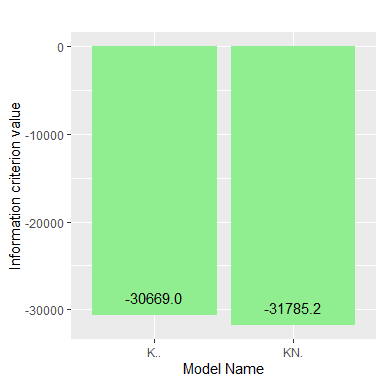### Thresholds objectively inferred from the clustering solution

The thresholds, calculated based on the estimated shape parameters, are provided in the output:

threshold_points <- threshold_out$optimal_model_results$thresholds
threshold_points$threholds #> Patient 1 Patient 2 Patient 3 Patient 4 #> 1 0.277 0.267 0.201 0.207 #> 2 0.747 0.772 0.769 0.814 ### Plotting the density estimates of the clustering solution The clustering solution can be visualized using the plot() function. The fitted and kernel density estimates, uncertainty and model selection plots can be obtained using the what parameter in this function. To generate the fitted and kernel density estimates, the data that has been used for analysis in the previous section, needs to be passed as an input using the data argument. Apart from static plots using plot_type = "ggplot" interactive plots are also available using plot_type = "plotly". The fitted densities and the estimated mixing proportions are displayed in the plots. The thresholds for the methylation states can be displayed using threshold = TRUE. As the K$$\cdot\cdot$$ model constrains the shape parameters to be equal for each patient, a single pair of threshold points is calculated for all patients, and this results in the same fitted density for all patients. In the KN$$\cdot$$ model, a pair of thresholds is independently determined for each patient based on the estimated shape parameters since the shape parameters differ for each patient. The parameter patient_number can be used to choose the patient for whom the fitted density and thresholds need to be visualized. For visualization, the parameter patient_number accepts the patient’s column number as a value. The fitted density and threshold points for the first patient in the dataset can be visualized as shown below: plot(threshold_out, what = "fitted density", threshold = TRUE, data = pca.methylation.data[,2:5], patient_number = 1, plot_type = "ggplot")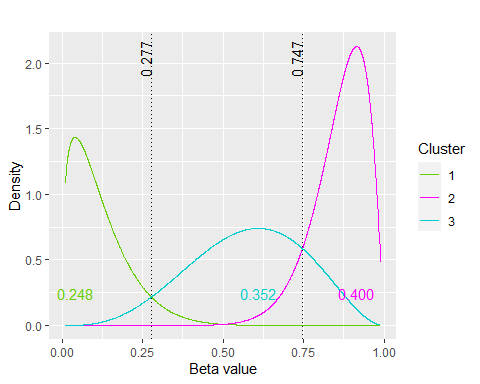The kernel density estimates for the first patient are displayed as below: plot(threshold_out, what = "kernel density", threshold = TRUE, data = pca.methylation.data[,2:5], plot_type = "ggplot")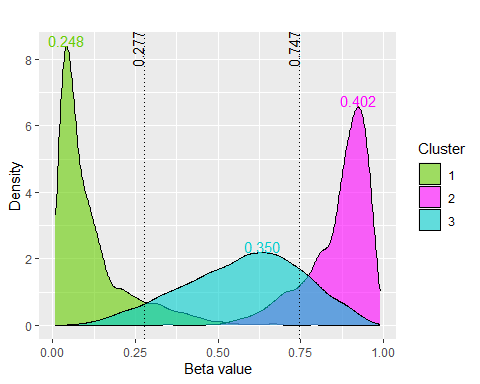### Plotting the uncertainties in the clustering solution The uncertainties in clustering represent the probability of a CpG site not belonging to the corresponding cluster. The value $$\hat{z}_{ck}$$ is the conditional probability that the CpG site c belongs to the cluster k. For each CpG site c, $$(1- \max_k {\hat{z}_{ck}})$$ is the measure of uncertainty in the associated membership. A low uncertainty shows good certainty in the clustering of the CpG site. A boxplot of the uncertainties in the clustering solution can be obtained as follows: plot(threshold_out, what = "uncertainty")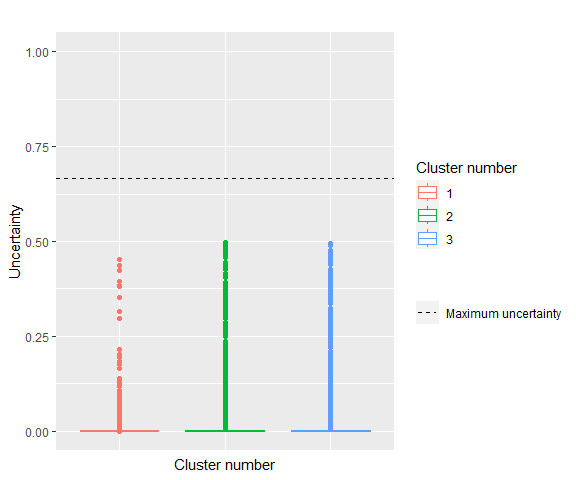## Identifying DMCs between R = 2 samples The methylation values in multiple samples can be analysed and the most differentially methylated CpG sites between these samples can be identified. The K$$\cdot$$R model (Majumdar et al. 2022) clusters the $$C \times (NR)$$ data into K = MR groups. A total of R = 2 DNA samples were collected from each patient (Silva et al. 2020). Each DNA sample has CpG sites that belong to one of the M methylation states, typically M = 3. As a result, the C CpG sites are clustered into K = MR = 9 biologically motivated groups. M <- 3 ## No. of methylation states in a DNA sample N <- 4 ## No. of patients R <- 2 ## No. of DNA samples my.seed <- 190 ## set seed for reproducibility dmc_output <- betaclust(pca.methylation.data[,2:9], M, N, R, model_names = "K.R", parallel_process = FALSE, seed = my.seed) #> fitting ... #> | | | 0% | |======================================================================| 100% ### AUC and WD metrics for finding similarities in the R cumulative distributions in each of the K clusters The area-under-curve (AUC) and the Wasserstein distance (WD) methods are used to compute the disparity between cumulative distributions, and are also utilised to quantify the degree of differential methylation of CpG sites between sample types in each cluster. The clusters are presented in descending order of their degree of differential methylation, based on decreasing AUC and, in the case of ties, WD values. print(dmc_output$optimal_model_results$DM$AUC)
#>  0.756771 0.682717 0.674868 0.580724 0.574759 0.568218 0.548141 0.543721
#>  0.529807
print(dmc_output$optimal_model_results$DM$WD) #>  0.149941623 0.064478308 0.070235457 0.022517255 0.033367011 0.006129221 #>  0.042324046 0.007467650 0.001898326 ### Summary statistics of the clustering solution Summary statistics of the clustering solution can then be obtained as follows: summary(dmc_output) #> ------------------------------------------------------ #> Multivariate beta mixture model fitted by EM algorithm #> ------------------------------------------------------ #> betaclust K.R model with 9 components: #> log-likelihood Information-criterion IC-value CpG-sites Patients Sample types #> 45040.33 BIC -89961.24 5067 4 2 #> #> Clustering table: #> 1 2 3 4 5 6 7 8 9 #> 403 828 257 923 278 647 798 458 475 #> #> Estimated mixing proportions: #> 0.082 0.156 0.064 0.177 0.054 0.127 0.157 0.09 0.094 ### Plotting the density estimates of the clustering solution The fitted density estimates of the clustering solution can be visualised. The names of the DNA samples used in the analysis are passed to the function using sample_name. If no input is provided in sample_name then default values of sample names, for e.g. Sample 1, Sample 2, etc. are used. The estimated mixing proportions are also displayed in each panel. plot(dmc_output, what = "fitted density", plot_type = "ggplot", data = pca.methylation.data[,2:9], sample_name = c("Benign","Tumour"))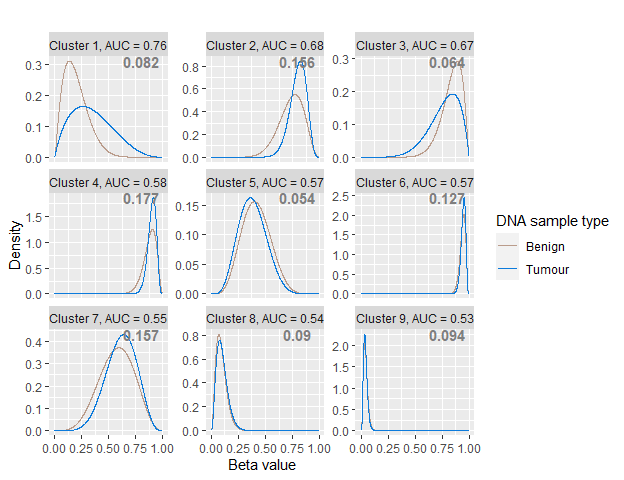The kernel density estimates of the clustering solution are obtained as below: plot(dmc_output, what = "kernel density", plot_type = "ggplot", data = pca.methylation.data[,2:9])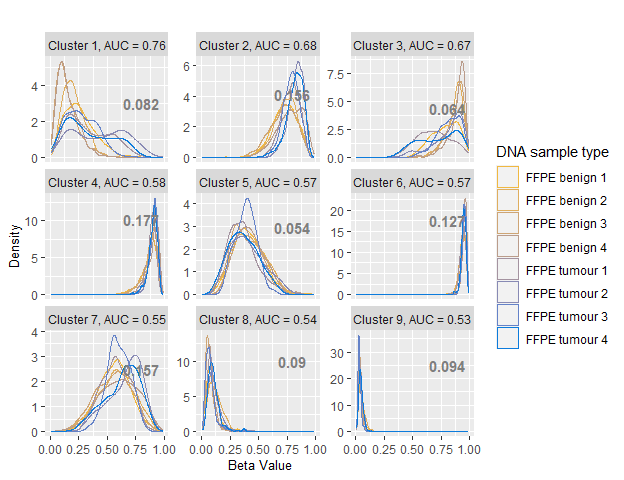### Plotting the uncertainty in the clustering solution The uncertainties in the clustering solution can be plotted as follows: plot(dmc_output, what = "uncertainty", plot_type = "ggplot")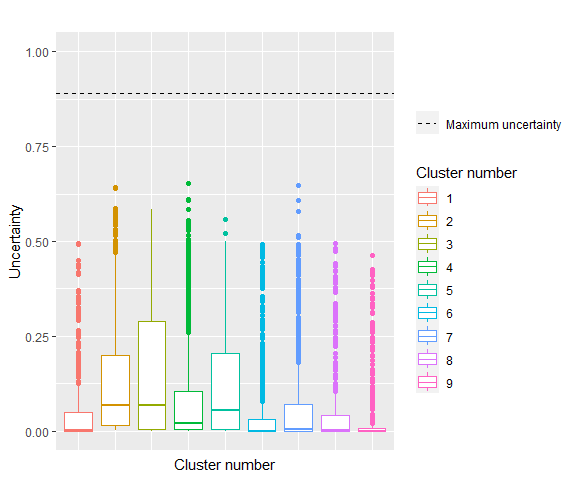#### Retrieving the most differentially methylated CpG sites The AUC and WD metric along with the density estimates suggests cluster 1, 2 and 3 as the mostly differentially methylated clusters. We then use the function DMC_identification() and pass the value of 0.65 to the parameter threshold and the value “AUC” to the parameter metric. The function then selects the clusters having AUC value higher than the value mentioned and retrieves the CpG sites clustered in those clusters as the most differentially methylated CpG sites.  ## the dataframe containing methylation data for the CpG sites identified as the most differentially methylated ones dmc_df <- DMC_identification(dmc_output, data = pca.methylation.data[,2:9], pca.methylation.data[,1], threshold = 0.06, metric="WD") ### Plotting the empirical cumulative distribution function Hypermethylation of RARB genes is an important biomarker in prostate cancer studies (Ameri et al. 2011). The MethylationEPIC annotated data (see help("legacy.data")) available in the package betaclust can be used to obtain information on the genes to which the selected DMCs are related. The differentially methylated CpG sites related to RARB genes are selected and passed to the ecdf.betaclust() function to visualise the empirical distribution functions (see help("ecdf.betaclust")). From the plot it is visible that the CpG sites in the tumour samples have increased beta values compared to those in the benign samples, suggesting hypermethylation of the CpG sites.  ##read the legacy data data(legacy.data) head(legacy.data) #> IlmnID Genome_Build CHR MAPINFO UCSC_RefGene_Name #> 513270 cg00008971 37 1 45966925 MMACHC;CCDC163P #> 322690 cg00032544 37 11 119680597 #> 575041 cg00034300 37 3 171496417 PLD1;PLD1 #> 718171 cg00035869 37 6 170462469 #> 147570 cg00037223 37 16 29821824 MAZ;MAZ #> 7716 cg00039998 37 8 133069361 OC90 #> UCSC_CpG_Islands_Name #> 513270 chr1:45965586-45966049 #> 322690 #> 575041 #> 718171 #> 147570 chr16:29823163-29823906 #> 7716 ## create empty dataframes and matrices to store the DMCs related to the RARB genes ecdf_rarb <- data.frame() cpg_rarb <- data.frame(matrix(NA, nrow = 0, ncol = 6)) colnames(cpg_rarb) <- colnames(legacy.data) ## split the UCSC_RefGene_name column to read the gene name related to that CpG site ## select the CpG sites related to the RARB genes a <- 1 for(i in 1:nrow(legacy.data)) { str_vec = unlist(strsplit(as.character(legacy.data[i,"UCSC_RefGene_Name"]),"[;]")) if(length(str_vec) != 0) { if(str_vec[] == "RARB") { cpg_rarb[a,] <- legacy.data[i,] a <- a+1 } } } ## Read the DMCs related to the RARB genes ecdf_rarb <- dmc_df[dmc_df$IlmnID %in% cpg_rarb\$IlmnID,]

## Plot the ecdf of the selected DMCs
ecdf.betaclust(ecdf_rarb[,2:9], R = 2, sample_name = c("Benign","Tumour"))